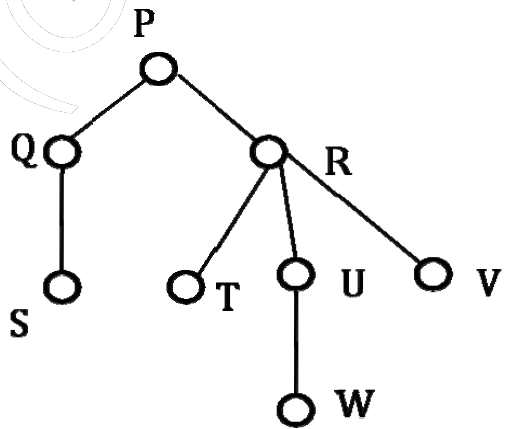# GATE | GATE-CS-2014-(Set-3) | Question 65

• Last Updated : 28 Jun, 2021

Consider the following rooted tree with the vertex P labeled as rootThe order in which the nodes are visited during in-order traversal is
(A) SQPTRWUV
(B) SQPTURWV
(C) SQPTWUVR
(D) SQPTRUWV

Explanation:

```Algorithm Inorder(tree) - Use of Recursion
Steps:
1. Traverse the left subtree,
i.e., call Inorder(left-subtree)
2. Visit the root.
3. Traverse the right subtree,
i.e., call Inorder(right-subtree)

Understanding this algorithm requires the basic
understanding of Recursion

Therefore, We begin in the above tree with root as
the starting point, which is P.

# Step 1( for node P) :
Traverse the left subtree of node or root P.
So we have node Q on left of P.

-> Step 1( for node Q)
Traverse the left subtree of node Q.
So we have node S on left of Q.

* Step 1 (for node S)
Now again traverse the left subtree of node S which is
NULL here.

* Step 2(for node S)
Visit the node S, i.e print node S as the 1st element of
inorder traversal.

* Step 3(for node S)
Traverse the right subtree of node S.
Which is NULL here.

Now move up in the tree to Q which is parent
of S.( Recursion, function of Q called for function of S).
Hence we go back to Q.

-> Step 2( for node Q):
Visit the node Q, i.e print node Q as the 2nd
element of inorder traversal.

-> Step 3 (for node Q)
Traverse the right subtree of node Q.
Which is NULL here.

Now move up in the tree to P which is parent
of Q.( Recursion, function of P called for function of Q).
Hence we go back to P.

# Step 2(for node P)
Visit the node P, i.e print node S as the 3rd
element of inorder traversal.

# Step 3 (for node P)
Traverse the right subtree of node P.
Node R is at the right of P.

Till now we have printed SQP as the inorder of the tree.
Similarly other elements can be obtained by traversing
the right subtree of P.

The final correct order of Inorder traversal would
be SQPTRWUV. ```
My Personal Notes arrow_drop_up